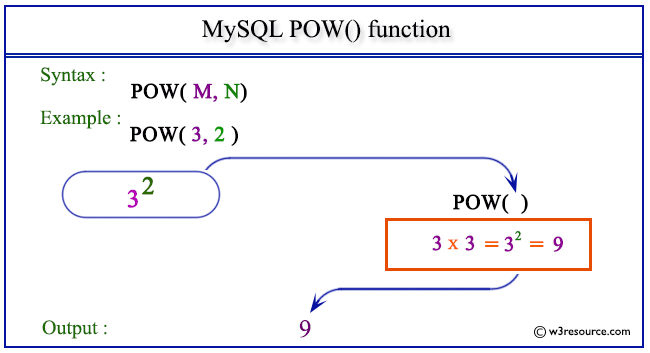# MySQL POW() function

## POW() function

MySQL POW() returns the value of a number raised to the power of another number. The synonym of POW() is POWER().

Syntax:

```POW(M,N);
```

Arguments

Name Description
M A number which is the base of the exponentiation.
N A number which is the exponent of the exponentiation.

Syntax Diagram:MySQL Version: 5.6

Pictorial presentation of MySQL POW() functionExample of MySQL POW() function

Code:

``````SELECT POW(3, 2);
```
```

Explanation

The above MySQL statement returns the value of 32, i.e. 9.

Sample Output:

```mysql> SELECT POW(3, 2);
+-----------+
| POW(3, 2) |
+-----------+
|         9 |
+-----------+
1 row in set (0.04 sec)
```

Example: POW() function with negative value

Code:

``````SELECT POW(4,-2);
```
```

Explanation

The above MySQL statement returns the value of 4-2, i.e. 0.0625.

Sample Output:

```mysql> SELECT POW(4,-2);
+-----------+
| POW(4,-2) |
+-----------+
|    0.0625 |
+-----------+
1 row in set (0.00 sec)
```

All Mathematical FunctionsPrevious: PI()
Next: POWER()

﻿